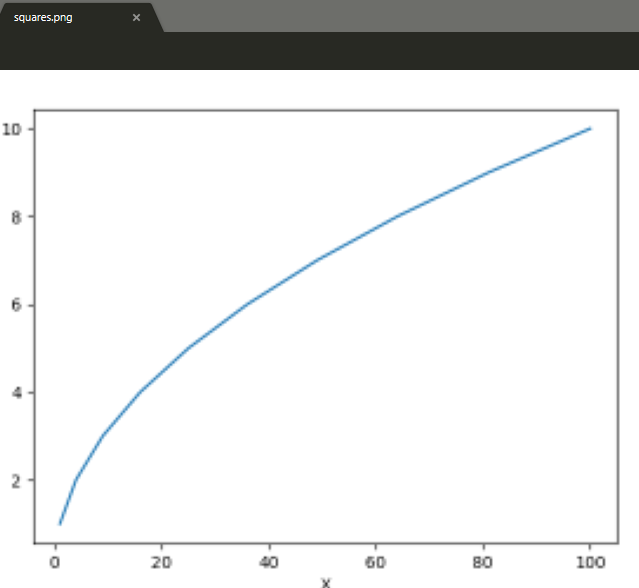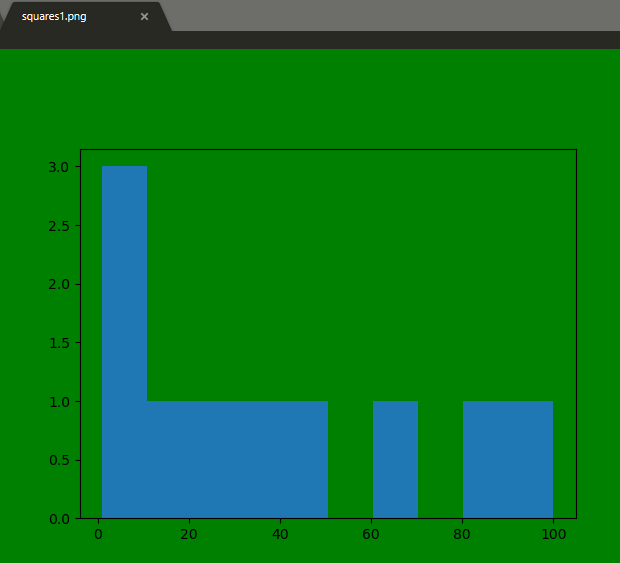# Matplotlib.pyplot.savefig() in Python

## Matplotlib.pyplot.savefig() in Python

Matplotlib is highly useful visualization library in Python. It is a multi-platform data visualization library built on NumPy arrays and designed to work with the broader SciPy stack. Visualization plays a very important role as it helps us to understand huge chunks of data and extract knowledge.

## Matplotlib.pyplot.savefig()

As the name suggests savefig() method is used to save the figure created after plotting data. The figure created can be saved to our local machines by using this method.

Syntax: savefig(fname, dpi=None, facecolor=’w’, edgecolor=’w’, orientation=’portrait’, papertype=None, format=None, transparent=False, bbox_inches=None, pad_inches=0.1, frameon=None, metadata=None)

Parameters:

PARAMETERS DESCRIPTION
fname Filename .png for image, .pdf for pdf format.
File location can also be specified here.
dpi Number of dots per inch.(picture quality)
papertype Paper type could be “a0 to a10”, “executive”,
“b0 to b10”, “letter”, “legal”, “ledger”.
format File format such as .png, .pdf.
facecolor and edgecolor Default as White.
bbox_inches Set it as “tight” for proper fit of the saved figure.
transparent Makes background of the picture transparent.
Orientation Landscape or Portrait.

Example 1:

 `# importing required modules ` `import` `matplotlib.pyplot as plt` ` ` `# creating plotting data` `xaxis ``=``[``1``, ``4``, ``9``, ``16``, ``25``, ``36``, ``49``, ``64``, ``81``, ``100``]` `yaxis ``=``[``1``, ``2``, ``3``, ``4``, ``5``, ``6``, ``7``, ``8``, ``9``, ``10``]` ` ` `# plotting ` `plt.plot(xaxis, yaxis)` `plt.xlabel(``"X"``)` `plt.ylabel(``"Y"``)` ` ` `# saving the file.Make sure you ` `# use savefig() before show().` `plt.savefig(``"squares.png"``)` ` ` `plt.show()`

Output :Example 2:

 `# importing the modules ` `import` `matplotlib.pyplot as plt` ` ` ` ` `# creating data and plotting a histogram` `x ``=``[``1``, ``4``, ``9``, ``16``, ``25``, ``36``, ``49``, ``64``, ``81``, ``100``]` `plt.hist(x)` ` ` `# saving the figure.` `plt.savefig(``"squares1.png"``,` `            ``bbox_inches ``=``"tight"``,` `            ``pad_inches ``=` `1``,` `            ``transparent ``=` `True``,` `            ``facecolor ``=``"g"``,` `            ``edgecolor ``=``'w'``,` `            ``orientation ``=``'landscape'``)` ` ` `plt.show()`

Output :Last Updated on March 17, 2022 by admin

## Conway’s Game Of Life (Python Implementation)Conway’s Game Of Life (Python Implementation)

Conway’s Game Of Life (Python Implementation) Conways’s Game Of Life is a Cellular Automation Method

## Python program to get all subsets of given size of a setPython program to get all subsets of given size of a set

Python program to get all subsets of given size of a set Given a set,

## How to read multiple text files from folder in Python?How to read multiple text files from folder in Python?

How to read multiple text files from folder in Python? Python is a strong language

## Find the length of a set in PythonFind the length of a set in Python

Find the length of a set in Python In Python, a Set is a collection data type that

## Python String isspace() MethodPython String isspace() Method

Python String isspace() Method Python String isspace() is a built-in method used for string handling. The isspace()

## Create a Snake-Game using Turtle in PythonCreate a Snake-Game using Turtle in Python

Create a Snake-Game using Turtle in Python A snake game is an arcade maze game which has

## How to install requests in Python – For windows, linux, macHow to install requests in Python – For windows, linux, mac

How to install requests in Python – For windows, linux, mac Requests is an elegant

## matplotlib.pyplot.scatter() in Pythonmatplotlib.pyplot.scatter() in Python

matplotlib.pyplot.scatter() in Python Matplotlib is a comprehensive library for creating static, animated, and interactive visualizations in